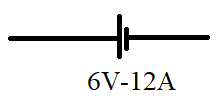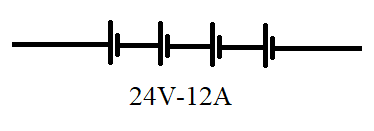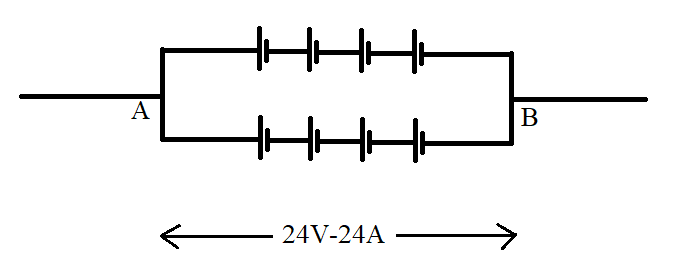Courses
Courses for Kids
Free study material
Offline Centres
MoreLast updated date: 04th Dec 2023
Total views: 281.7k
Views today: 3.81k

# How many cells each marked $6V - 12A$ should be connected in mixed grouping so that he marked $24V - 24A$ $A.$ $4$ $B.$ $8$ $C.$ $12$ $D.$ $6$Verified
281.7k+ views
Hint: In this problem the cell which is having $emf$ of $6V$ and current of $12A$ is connected in different combinations that is series and parallel combination to obtain the $emf$ of $24V$ and current of $24A$ .

Complete step-by-step solution:
If the cells are connected in series the resultant $emf$ of the battery will be equal to sum of the $emf$ of the individual cell and the current remains same .If $E$ is the resultant $emf$ of the of the battery with $n$ number of cells and ${E_1},{E_2},{E_3},{E_4}.......{E_n}.$ are the $emf$ of individual cells. Then
$E = {E_1} + {E_2} + {E_3} + {E_4}....... + {E_n}.$……… $\left( 1 \right)$
$I$ remains the same.
If the cells are connected in parallel the resultant current of the battery will be equal to sum of the current of the individual cell and the $emf$ remains same .If $I$ is the resultant current of the of the battery with $n$ number of cells and ${I_1},{I_2},{I_3},{I_4}.......{I_n}.$ are the current of individual cells. Then
$I = {I_1},{I_2},{I_3},{I_4}.......{I_n}.$ ……… $\left( 2 \right)$
$E$ remains the same.

Given:
$\Rightarrow$ Each cell have $emf$ of $6V$ and current of $12A$
$\varepsilon = 6V,I = 12A$This cell should be connected to get resultant $emf$ of $24V$ and current of $24A$
$\Rightarrow$ When $4$ cells are connected in series the resultant $emf$ is $24V$ and the current remains the same that is $12A$ because the current is same in series combination
$\varepsilon = 24V,I = 12A$$\Rightarrow$ Then this combination is connected in parallel the resultant $emf$ remains the same that is $24V$ because voltage or $emf$ remains same in parallel combination and the current will be $24A$
$\varepsilon = 24V,I = 24A$Therefore, $8$ cells are connected in mixed group as shown above to get, $\varepsilon = 24V,I = 24A$
Hence, option $B$ is correct. That is $8$

Note: In series connection the current will be the same and potential difference or voltage or $emf$ will be different. Whereas in parallel combination the current will be different and the potential difference will be the same.